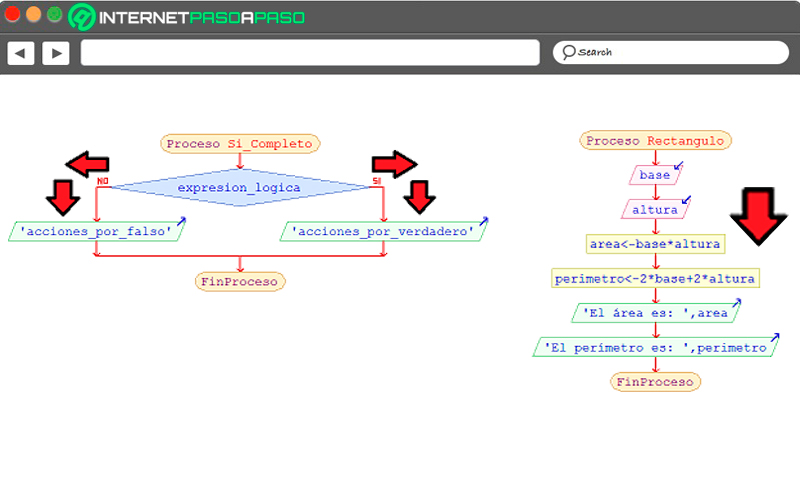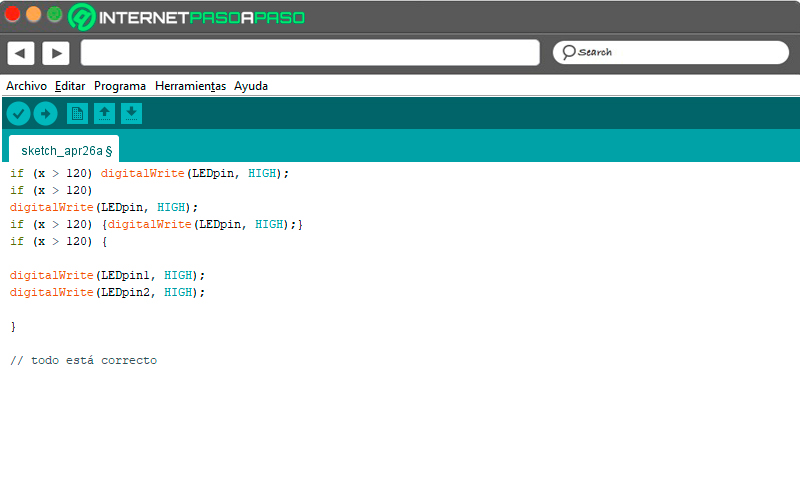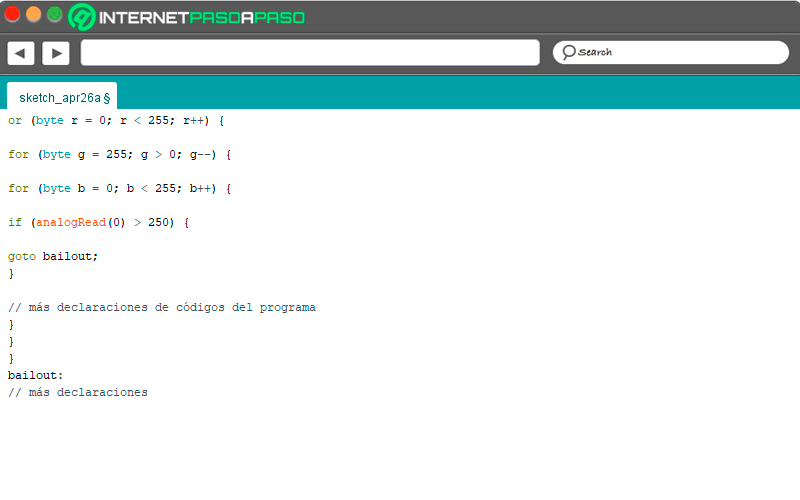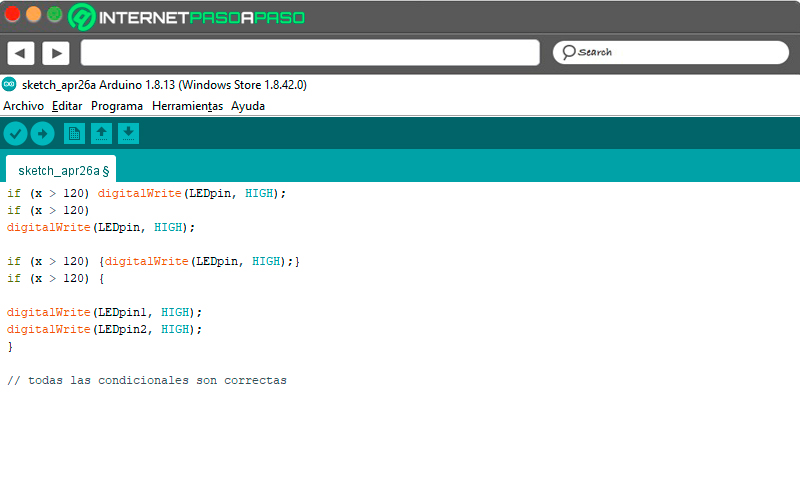# Control Structures in Arduino  What are they? + Rates ▷ 2021

The control structures within the Arduino IDE will help you create the programs for your projects with the Arduino board. For this reason, it is important that you know the secrets of this programming language tool, which we will show you below.

The first thing we will do is analyze the different types of control structures in Arduino and understand why they are so important to carry out the sketch.

Also we will mention the other structures in the Arduino programming that you must know to develop your own projects from scratch. Pay attention to every detail to become a true electronics expert.

## What are control structures and what are they for in Arduino project programming?The control structures in the Arduino programming environment are tools that are used to choose a sequence or an alternative path within the structure of the software. This means that, They are instructions that allow breaking the methodical sequence of the codes, since there is a logical expression with which to decide if one route or the other is chosen so that both can later reach the same end of the process.

To get one better administration and reading of the programming code, it is necessary to correctly use the tabulation in each structure. This will help to find errors or sequences to be repeated faster.

## Types of control structures in Arduino What are all that exist?

There are two types of control structure within Arduino programming, which are:

### Decision structuresThis type of structure refers to the path that the program must take when there is a variable that divides the sequence of the codes in two. To do this, a condition is evaluated and returns a value (true or false) to make the decision of which instructions to execute, depending on the response.

In Arduino you will find the following conditional statements:

### if

You shall use this tool when you want to know if a variable reached a certain condition.

Su syntax is:

```if (condition) { // Siendo condition una expresión de TRUE o FALSE.

//statement(s)

}
```

For example:

```if (x > 120) digitalWrite(LEDpin, HIGH);

if (x > 120)

digitalWrite(LEDpin, HIGH);

if (x > 120) {digitalWrite(LEDpin, HIGH);}

if (x > 120) {

digitalWrite(LEDpin1, HIGH);

digitalWrite(LEDpin2, HIGH);

}

// todo está correcto
```

In case of adding else, then what is sought is that, if it does not meet a conditionIn this way, the program must carry out a specific action.

### else

With this control structure tool multiple conditions can be chosen that are mutually exclusive when a certain situation occurs.

It has to be used with the following structure:

```if (condition1) {

// do Thing A

}

else if (condition2) {

// do Thing B

}

else {

// do Thing C

}```

### switch – case

You can use this structure when you need the program to decide on a precise instruction when there is the possibility of comparing the value of the variable in relation to previously established values.

Its syntax is set as follows:

```switch (var) {

case label1:

// statements

break;

case label2:

// statements

break;

default:

// statements

break;

}
```

Where var is the variable that the program has to compare with other data (provided they are of the type int Y char). Y label are the constants executed. It’s used break to exit the switch and default to execute the code block when the requested conditions are not met.

### Repetition structuresThese loop structures They are tools that allow you to execute the instructions that the program has repeatedly.

Among the most used sentences in Arduino are:

### for

You will be able to repeat the sequences of the codes that are between the keys the times that you indicate in the Arduino IDE. To do this, it will be analyzed that the conditions are met for this series of control structures to be executed again.

It is developed following these parameters:

```for (initialization; condition; increment) {

// statement(s);

}
```

Where initialization it means that it will be once and at the beginning of the structure. Condition is the sentence that must occur in the program to enter the loop in question (keep in mind that the loop will end when it delivers the condition FALSE). In case the loop throws TRUE, then it will be executed by means of the increase.

### while

With this tool you can run the loop continuously and as many times as necessary, as long as the condition set at the beginning of the loop in parentheses is met. If it returns a false value, the expression is exited and the action ends.

Its structure is:

```while (condition) { // la condición debe ser TRUE o FALSE

// statement(s)

}```

### do while

This loop is used in the same way as the earlier while statement. But in this case the condition for repeating the structure is at the end of the block, enclosed in parentheses.

Its syntax is:

```do {

// statement block

} while (condition);
```

An example of this is:

```int x = 0;

do {

delay(50); // para esperar que se estabilice el sensor

x = readSensors(); // lectura de los sensores

} while (x < 100);
```

### goto

This tool is very useful when the program needs to be transferred to a certain point, according to the previously established label.

For example:

```for (byte r = 0; r < 255; r++) {

for (byte g = 255; g > 0; g--) {

for (byte b = 0; b < 255; b++) {

goto bailout;

}

// más declaraciones de códigos del programa

}

}

}

bailout:

// más declaraciones
```

### break

When you need to get out of the control structure you will have to use this statement. Must be use with do, for or while.

An example code is:

```int threshold = 40;

for (int x = 0; x < 255; x++) {

analogWrite(PWMpin, x);

if (sens > threshold) { // rescatar en la detección del sensor

x = 0;

break;

}

delay(50);

}```

### continue

To skip the rest of the sequences captured in the program, it is necessary to use this tool together with do, for or while.

For example:

```for (int x = 0; x <= 255; x ++) {

if (x > 40 && x < 120) { // crear salto en los valores

continue;

}

analogWrite(PWMpin, x);

delay(50);

}```

## Other structures in Arduino programming that you should know to develop your own projectsIn addition to the structures mentioned above, there are other tools that should also be taken into account when programming your Arduino board.

See below:

### Sketch

The sketch, also known as arduino sketch, is the programming structure in which they are included all the codes that allow the project to be carried out so that the board can carry out the desired actions. It is composed of variables, functions added by operator, setup () and loop () tools, and comments made by the programmer.

Within this programming environment it is possible to find a message area to show the errors or the correct operation of the program. The toolbar is also used to access the different commands. It is important to mention that the length of a sketch is .ino and for the sketch to work it is necessary that the directory has the same name as the sketch, although it is possible that the sketch is in another file, but it must belong to a directory with the same name.

The structure of the sketch is:

```void setup() {

// agrega tu código de configuración aquí para ejecutar una vez:

}

void loop() {

// ingresa el código principal aquí para que se ejecute de forma repetida:

}```

### Arithmetic operators

The Arithmetic operators are symbols that are used within programming of an Arduino software to include functions of Addition, subtraction, multiplication and division. Keep in mind that the returned values ​​are based on the data that has been defined in the operands, for example, int (in this case the results obtained will not have decimals).

Taking into account the above, it is possible to propose the following example:

```int a = 5;

int b = 10;

int c = 0;

c = a + b; // la variable c obtiene un valor de 15 después de que se ejecuta esta declaración
```

If for some reason the operands are of different types, program will take the most important operand and return a value based on that criteria. For example, if there are 2 data (one of type int and the other float, being this greater) the C ++ will return a result in floating point.

The following example follows:

```float a = 5.5;

float b = 6.6;

int c = 0;

c = a + b; // la variable 'c' almacena un valor de 12 solo en contraposición a la suma esperada de 12.1
```

### Comparison operators

Like the arithmetic operators, in this group are include symbols that aid in better programming, but taking into account a conditional variable.

Therefore, it is possible to find in this set the following symbols:

```x == y (la variable x es igual a y). Hay que tener presente que se usa el doble igual (==), ya que un solo = representará otra asignación.

x! = y (la variable x no es igual a y)

x < y (la variable x es menor que y)

x > y (la variable x es mayor que y)

x < = y (la variable x es menor o igual que y)

x > = y (la variable x es mayor o igual que y)
```

An example of a programming structure with comparison operators is:

```if (x > 120) digitalWrite(LEDpin, HIGH);

if (x > 120)

digitalWrite(LEDpin, HIGH);

if (x > 120) {digitalWrite(LEDpin, HIGH);}

if (x > 120) {

digitalWrite(LEDpin1, HIGH);

digitalWrite(LEDpin2, HIGH);

}

// todas las condicionales son correctas
```

If you have any questions, leave them in the comments, we will answer you as soon as possible, and it will also be of great help to more members of the community. Thanks! 😉##### Author: Félix Albornoz

I have been working in the technology sector for more than 20 years helping companies and users to develop and train in this field. Always learning new things.